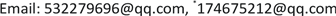1海军工程大学动力工程学院，湖北 武汉

2国防科技大学信息通信学院，湖北 武汉1. 引言

2. 燃气轮机自适应控制

Y = f ( X , U i n , U a m ) (1-1)

M = X a c t X c a l (1-2)

Y = f ( X , U i n , U a m ) (1-3)

F = ∑ i = 1 n 1 ( C a i − C c i ) 2 + ∑ i = 1 n 2 ( e r r i ) 2 (1-4)

3. 最优算法的构造3.1. 灰度预测

x ( 0 ) ( k + 1 ) = a − 1 2 ( x ( 1 ) ( k ) + x ( 1 ) ( k + 1 ) ) + u (2-1)

(2-2)

X ( 1 ) = { x ( 1 ) ( 1 ) , x ( 1 ) ( 2 ) , ⋯ ⋯ , x ( 1 ) ( n ) } (2-3)

(2-4)

∅ = [ a , u ] T 为待辨识参数向量，则(2-1)可写成：

Y = B * ∅ (2-5)

x ^ ( 1 ) ( k + 1 ) = a x ( 1 ) ( k ) − u ^ a ^ e − a ^ k + u ^ a ^ (2-7)

x ^ ( 0 ) ( k + 1 ) = x ^ ( 1 ) ( k + 1 ) − x ^ ( 1 ) ( k ) = ( 1 − e a ^ ) x ^ ( 1 ) ( k ) − x ^ ( 1 ) e − a ^ k (2-8)

x ^ i ( 0 ) ( k + 1 ) 即为预估的部件修正因子 。取 ( ± 10 % + 1 ) M ^ i 为遗传算法中的种群空间进行进一步优化求解。

3.2. 自适应遗传算法(AGA)

P c = { K 1 f max − f ′ 1 f max − f avg , f ′ ≥ f avg K 3 , f ′ < f avg (2-9)

P m = { K 2 f max − f ′ 2 f max − f avg , f ′ ≥ f avg K 4 , f ′ < f avg (2-10)

fmax，群体中的最大适应值fmax，群体中的最大适应值，favg，群体平均适应值favg，群体平均适应值， f ′ 1 要交叉的两个个体中较大的适应值， f ′ 2 要变异个体的适应值，K1，K2，K3和K4是常数。

4. 燃气轮机数学模型精确解

3个输入参数分别为：环境温度T0、环境压力P0、燃油质量流量G2；1个平衡检验误差参数为：轴功率平衡误差e。

F = 1 1 + F c (3-1)

Modified factor

(3-1)式中 F c 由式(1-4)获得，由于式(1-4)中的定义可知函数 F c 为正数，随着Mi逐步优化， F c 逐步减少，适应度F逐步增大，适应度极值等于1。

Relative error between calculated value and measured value at design operating conditio

AGA算法GM−AGA算法
n00.699−0.299
G1−0.449−0.4260.314
T1−0.550−0.1330.194
P1−1.010−0.0665−0.217
T2−0.942−0.879−0.101
T3−1.357−0.857−0.604
T40.6040.541−0.142
P2−0.6280.5530.221
T5−1.036−0.2080.369
e0.3070.345−0.191
F c0.1440.04600.0224

5. 结束语